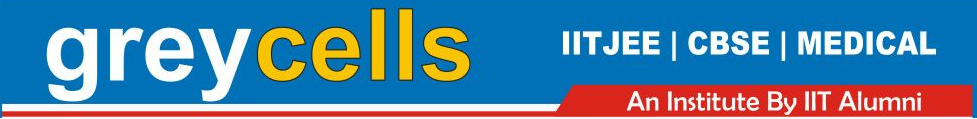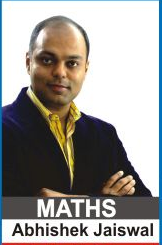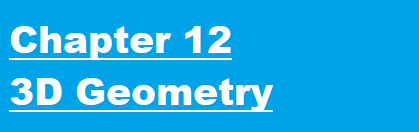Maths Lectures by Abhishek Jaiswal (B.Tech IIT Roorkee) for class 12th Students. The lectures cover theory both for CBSE and Competetive Examinations.

For Queries and Live online batches contact 9312922770.Lecture 1 | 3D Geometry - Introduction | Position Vectors

Lecture 2 | 3D Geometry - Straight Line in Space

Lecture 3 | 3D Geometry - Straight Line in Space

Lecture 4 | 3D Geometry - Straight Line | Plane in Space

Lecture 5 | 3D Geometry - Planes | Equation of Plane in Space

Lecture 6 | 3D Geometry - Planes in Space

Lecture 7 | 3D Geometry - Point & Plane | Line & Plane

Lecture 8 | 3D Geometry - Plane & a Plane | Line & a Plane

Lecture 9 | 3D Geometry - SPHERE